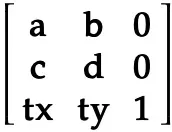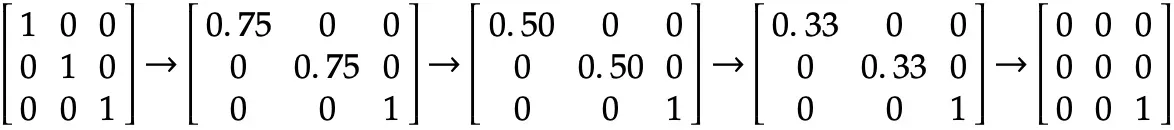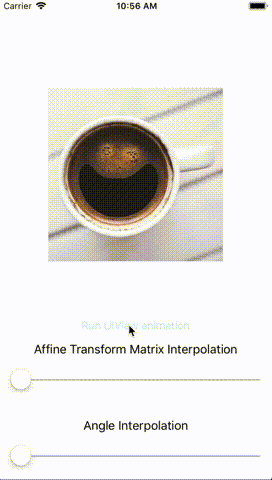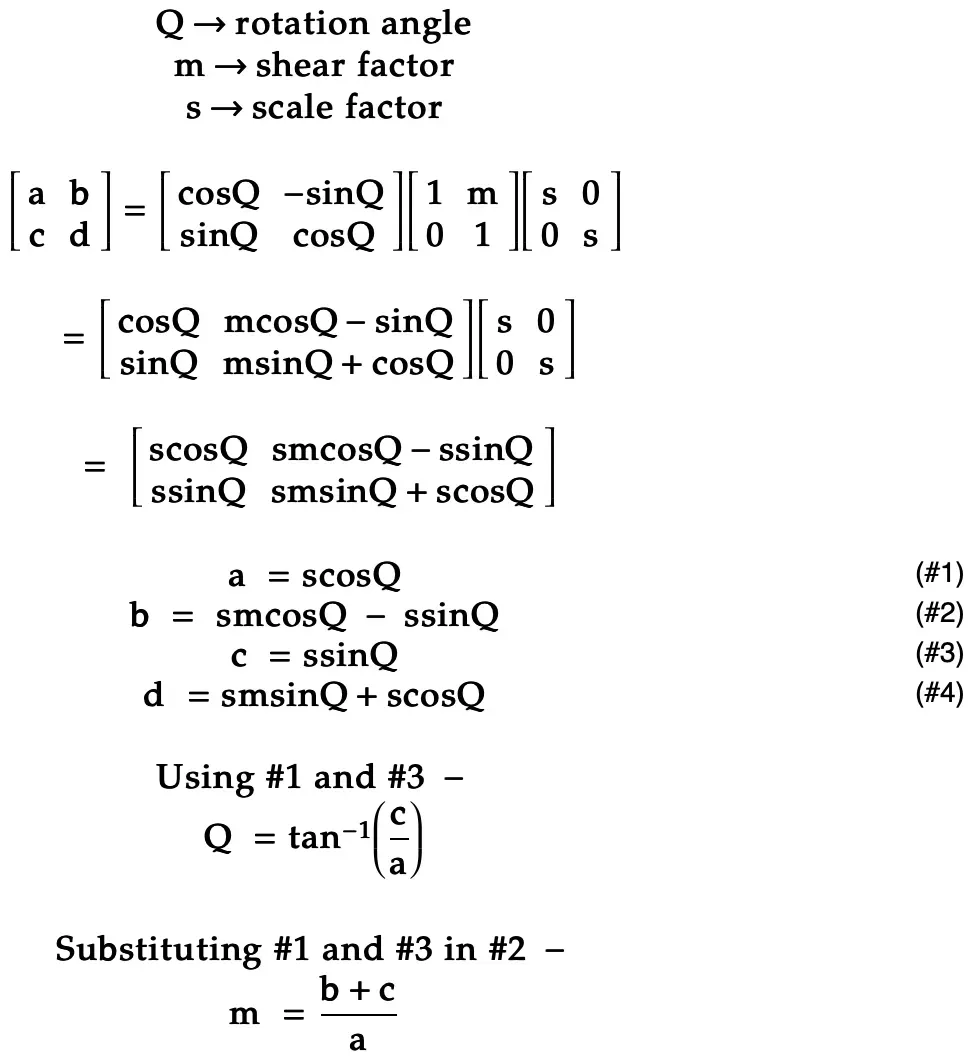# Debugging CGAffineTransform Scale to Zero Animations on iOS

I was recently working on an application which required me to display an animation which scales a `UIView` down to zero. The approach I took was to apply a `CGAffineTransform` (with scale factor set to zero) to the `UIView` in a `UIView.animate()` block. However, when I implemented this, the view did not animate but just disappeared from the UI. The problem turned out to be something which is not really obvious from the iOS docs and hence I decided to write up this post in the hope that it will help others.

This is the behaviour I wanted to implement:This is the code I initially wrote up to do this:

``````let someView = UIView()
let scaleTransform = CGAffineTransform(scaleX: 0, y: 0)
UIView.animate(withDuration: 3.0, animations: {
view.transform = scaleTransform
})
``````

Here is the actual result of the above code on iOS 12.0 (quick note — this seems to behave differently on different iOS versions):This is an intriguing problem since, based on the iOS docs, this should have worked. After digging into this by creating a sample app and reading up on some “Affine Transform” math, I was able to track down the root cause.

For the impatient, here is a quick explanation of the root cause and a potential solution (a more detailed explanation follows this):

1. To perform a `CGAffineTransform` animation, iOS first decomposes both, the `scaleTransform` matrix and the current `UIView` transform (lets call this `currentTransform` which in our case is the identity transform) into its constituents — scale, shear, rotate and translate.
2. It then interpolates over each of these values from the `currentTransform` to `scaleTransform`, internally creating a new `CGAffineTransform` for each animation frame.
3. Finally, it applies these generated transforms to the UIView at the required frame rate to display the animation.
4. The root cause of our problem lies with the math to decompose a `CGAffineTransform` matrix. If the scale factor is zero, the math involved tries to divide by zero (which is undefined / infinity) and, as a result, the iOS animation behaves unpredictably.

A potential solution (and the one I used in my implementation) was to use a really small but non-zero value for the scale factor (e.g. `0.001`) and then set the `UIView.isHidden = true` when the animation completes so that the view is completely hidden from the UI.

## Detailed explanation:

Let us now move on to a more detailed explanation on why iOS decomposes the `CGAffineTransform` matrix, the math used to do this and how that leads to a problem.

A quick recap of the `CGAffineTransform` matrix and `UIView.animate()` methods:

## CGAffineTransform matrix

The `CGAffineTransform` is a 3x3 matrix where `a`, `b`, `c`, `d` together define the scale, shear and rotation while `tx` and `ty` define translation. Of these, specifically, `a` and `d` play a part in defining the scale (refer below image). This is how a standard `CGAffineTransform` matrix looks like:By default, the `UIView.transform` property is set to an identity matrix (`CGAffineTransform.identity`) and applying any of scale / shear / rotate / translate operations basically involves multiplying this identity matrix with the required matrix.

## UIView.animate()

`UIView.animate()` interpolates values for the animated properties from the initial value (i.e. value before animation) to the final value and applies this to the UIView for each animation frame over the specified duration. For example, the following code will interpolate `x` and `y` from 0 to 10 and keep applying it to the `someView.center` property at the required frame rate so that the user sees the `someView` animate to the new position over a duration of 3 seconds.

``````let someView = UIView()
someView.center = CGPoint(x: 0, y: 0)
UIView.animate(withDuration: 3.0, animations: {
someView.center = CGPoint(x: 10, y: 10)
})
``````

With this information, consider the original (buggy) code snippet (which animates the `UIView.transform` property). At face value, it is easy to assume that the iOS animation will just interpolate the identity matrix `[[1,0,0], [0,1,0], [0,0,1]]` (`initialTransform`) to `[[0,0,0], [0,0,0], [0,0,1]]` (`scaleTransform`) by interpolating each value of the matrix. Something like:CGAffineTransform animation interpolation assumption

To test if that is what actually happens, I put together a sample iOS application. This application uses a `CGAffineTransform` to rotate a `UIView` (I used rotation in the sample since the problem with our assumption is clearly demonstrated with this). The sample application works as follows:

1. Tapping on the “Run UIView animation” button runs the iOS animation to rotate the image by 160 degrees by generating a `CGAffineTransform` rotation matrix and applying it in a `UIView` animation block. (The intention is to understand how iOS internally does this).
2. Moving “Affine Transform Matrix Interpolation” slider generates intermediate matrices by interpolating each value of the matrix (similar to the what we discussed in the assumption) from the identity transform to a 160 degree rotation transform and applies it to the image.
3. Moving the “Angle Interpolation” slider generates intermediate matrices by interpolating over the angle (0 to 160 degrees) and apples it to the image.

This is how the sample application works:Notice that the intermediate frames in the actual iOS animation generated by tapping the “Run UIView animation” button look the same as the ones generated by “Angle Interpolation” where as those generated by interpolating each value of the `CGAffineTransform` matrix looks different and is not the expected behaviour. For this reason, when animating the `UIView.transform` property, iOS actually: decomposes the initial `CGAffineTransform` into its components values (scale, shear, rotation, translation etc.), then interpolates over each of these values, constructs a new `CGAffineTransform` matrix for each animation frame and applies it to the `UIView.transform` property.

Now, to debug further, lets look at the math used for decomposing the `CGAffineTransform` matrix. The math below is for the case when we scale, shear and rotate (in that order). Also, we can ignore translation (tx, ty) for this example since those values do not impact scale / shear / rotate.This means, the iOS SDK needs to divide by `a` to calculate the rotation angle and the shear factor. Now, If `s` (i.e. scale) is zero, `a` becomes zero (refer #1 above) and produces undefined results. As a result, the matrix decomposition fails and the iOS animation system produces unpredictable results.

Now, that we have the root cause, let us move on to the solution. To display a animation where the `UIView` needs to be scaled to zero, I suggest the following:

1. Using a very small but non-zero scale factor when creating the final `CGAffineTransform` for the animation.
2. On completion of the animation, setting the `UIView.isHidden` property to `true` so the UIView is hidden from the user.

In my case, I was able to make the animation work as expected by using a scale factor of `0.001` (i.e. `CGAffineTransform(scaleX: 0.001, y: 0.001)`). To see how this looks in an iOS app, please check the “expected behaviour” GIF which is the very first image in this post.

Wrapping up: in this post, we discussed how the internal workings of `UIView.animation()` and the math to decompose a `CGAffineTransform` matrix together lead to the “scale to zero” animation to fail unpredictably on iOS. We also discussed a potential solution / work around for this problem.

Thanks for taking the time to read this post. Hope you found it interesting and that it helps others facing a similar problem.

Happy coding!

References: